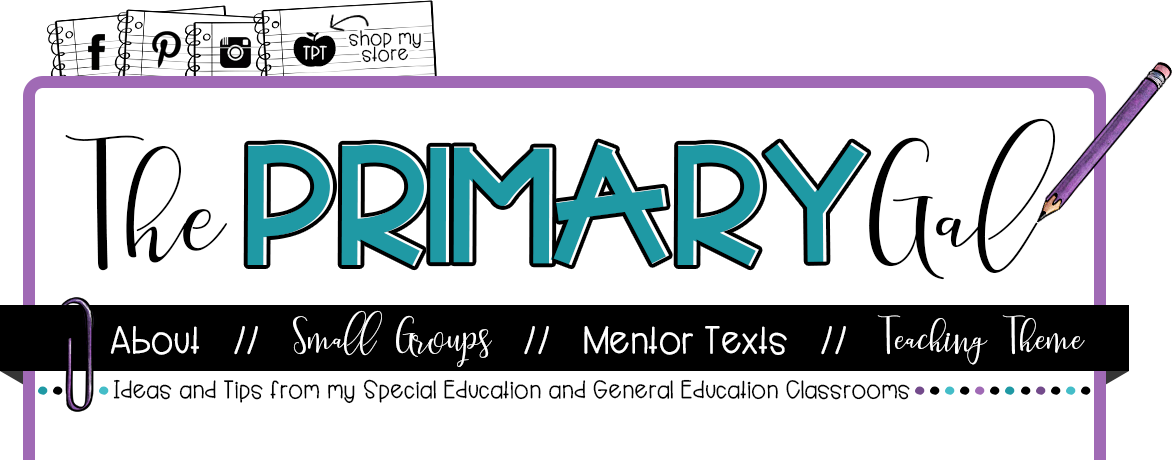-->### Area of a Triangle: Discovering the Formula

My area and perimeter unit is seriously one of my favorite things to teach in fifth!  I love the formulas, the real life math experiences, and the hands on learning that can happen.  This week, I did a simple, yet totally powerful demonstration to help my students discover, and most of all remember, the formula for the area of a triangle.

I gave each student a 5 inch by 5 inch square.  We used our rulers to measure five rows and five columns to show the area of our square.

Last year, I used a 4 inch by 4 inch square.  I am seriously struggling with which was the better alternative for this activity.  A 5x5 square will yield an area of a triangle with a decimal.  Since my kids were fairly comfortable with this, I went with it! :)

This is 100% review for my fifth graders, but that was also 100% the point!  I wanted my kids to see that finding the area of a triangle can be SUPER intimidating, but it is actually very similar to something that they are already experts at doing!

I had them lay their gridded square on their notebook.  Then, we wrote the formula area = length x width, and calculated the area.

Then, I said, "But hey....aren't we talking about the area of a TRIANGLES today?!  Errrr, that's embarrassing.  Can we somehow make this square into a triangle?"  <-----Unfortunately, I do say silly, sarcastic things like this!

They quickly discovered that by folding it in half, they could easily make a triangle.

They counted the squares and were able to calculate the area of the triangle without the use of a formula.

Then, I told them that I wanted to leave them alone for a while, and give them a chance to brainstorm a possible formula.  The lightbulbs were seriously going off like crazy!

After working through a few examples, and testing their possible formulas, we practiced finding the area of triangles with a game of Scoot!